Related Articles

# Decision Making in C / C++ (if , if..else, Nested if, if-else-if )

• Difficulty Level : Easy
• Last Updated : 30 Jun, 2021

There come situations in real life when we need to make some decisions and based on these decisions, we decide what should we do next. Similar situations arise in programming also where we need to make some decisions and based on these decisions we will execute the next block of code. For example, in C if x occurs then execute y else execute z. There can also be multiple conditions like in C if x occurs then execute p, else if condition y occurs execute q, else execute r. This condition of C else-if is one of the many ways of importing multiple conditions.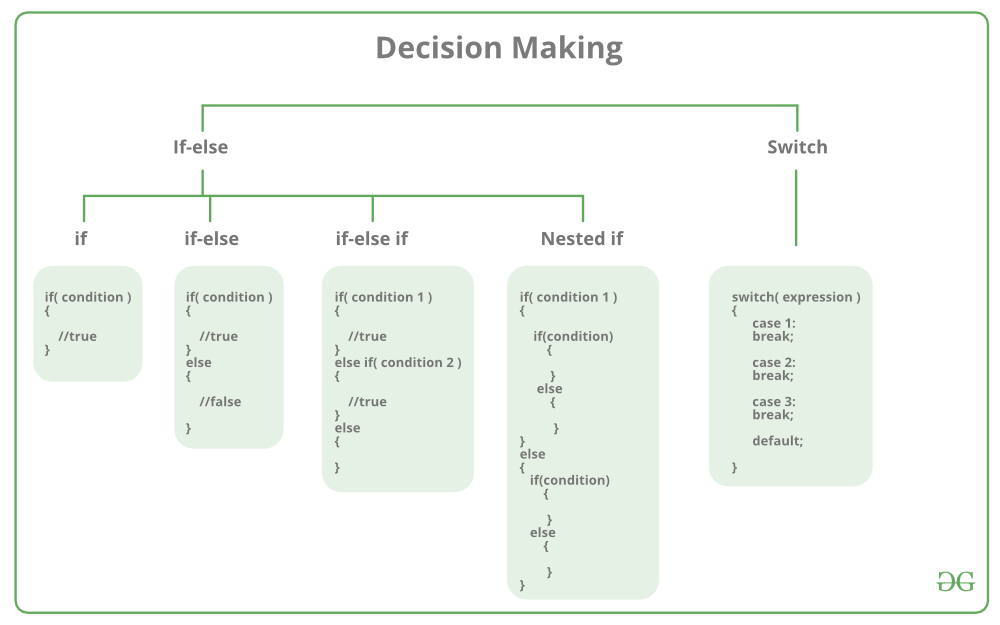Decision-making statements in programming languages decide the direction of the flow of program execution. Decision-making statements available in C or C++ are:

if statement in C/C++

if statement is the most simple decision-making statement. It is used to decide whether a certain statement or block of statements will be executed or not i.e if a certain condition is true then a block of statement is executed otherwise not.
Syntax

```if(condition)
{
// Statements to execute if
// condition is true
}```

Here, the condition after evaluation will be either true or false. C if statement accepts boolean values – if the value is true then it will execute the block of statements below it otherwise not. If we do not provide the curly braces ‘{‘ and ‘}’ after if(condition) then by default if statement will consider the first immediately below statement to be inside its block.
Example

```if(condition)
statement1;
statement2;

// Here if the condition is true, if block
// will consider only statement1 to be inside
// its block.```

Flowchart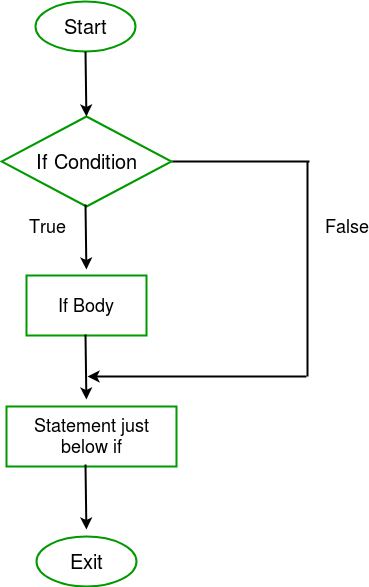## C

 `// C program to illustrate If statement``#include ` `int` `main() {``    ``int` `i = 10;` `    ``if` `(i > 15)``    ``{``       ``printf``(``"10 is less than 15"``);``    ``}   ``   ` `    ``printf``(``"I am Not in if"``);``}`

## C++

 `// C++ program to illustrate If statement``#include``using` `namespace` `std;` `    ``int` `main()``    ``{``        ``int` `i = 10;`` ` `        ``if` `(i > 15)``        ``{``           ``cout<<``"10 is less than 15"``;``        ``}   ``       ` `        ``cout<<``"I am Not in if"``;``    ``}`
Output:
`I am Not in if`

As the condition present in the if statement is false. So, the block below the if statement is not executed.

if-else in C/C++

The if statement alone tells us that if a condition is true it will execute a block of statements and if the condition is false it won’t. But what if we want to do something else if the condition is false. Here comes the C else statement. We can use the else statement with if statement to execute a block of code when the condition is false.
Syntax

```if (condition)
{
// Executes this block if
// condition is true
}
else
{
// Executes this block if
// condition is false
}```

Flowchart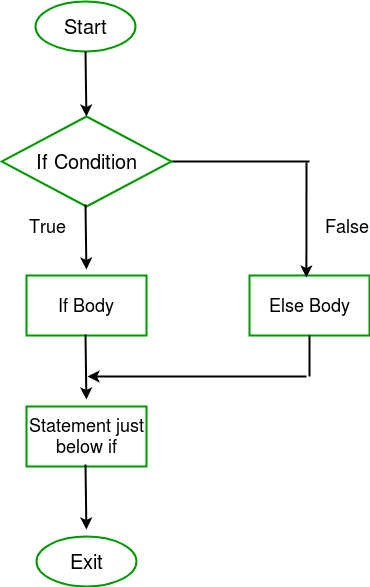Example:

## C

 `// C program to illustrate If statement``#include ` `int` `main() {``    ``int` `i = 20;` `    ``if` `(i < 15){``      ` `        ``printf``(``"i is smaller than 15"``);``    ``}``    ``else``{``      ` `        ``printf``(``"i is greater than 15"``);``    ``}       ``    ``return` `0;   ``}`

## C++

 `// C++ program to illustrate if-else statement``#include``using` `namespace` `std;` `int` `main()`` ``{``        ``int` `i = 20;`` ` `        ``if` `(i < 15)``            ``cout<<``"i is smaller than 15"``;``        ``else``            ``cout<<``"i is greater than 15"``;``            ` `    ``return` `0;   `` ``}`
Output:
`i is greater than 15`

The block of code following the else statement is executed as the condition present in the if statement is false.

nested-if in C/C++

A nested if in C is an if statement that is the target of another if statement. Nested if statements mean an if statement inside another if statement. Yes, both C and C++ allow us to nested if statements within if statements, i.e, we can place an if statement inside another if statement.
Syntax:

```if (condition1)
{
// Executes when condition1 is true
if (condition2)
{
// Executes when condition2 is true
}
}```

Flowchart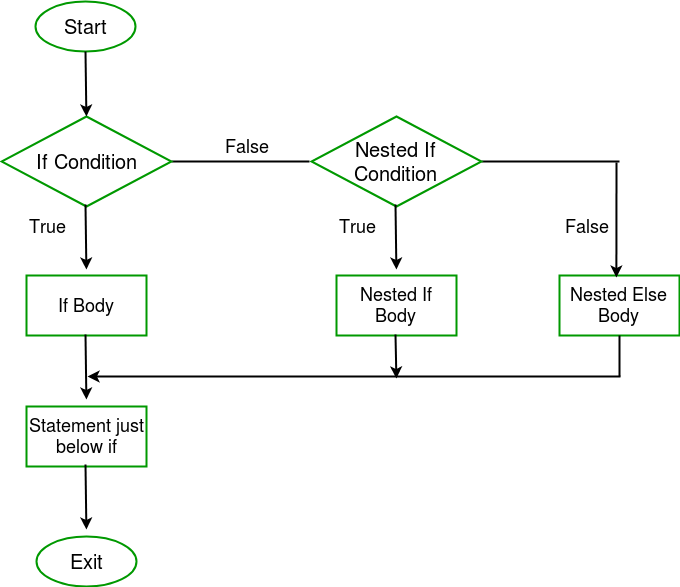Example:

## C

 `// C program to illustrate nested-if statement``#include ` `int` `main() {``    ``int` `i = 10;` `    ``if` `(i == 10)``    ``{``        ``// First if statement``        ``if` `(i < 15)``           ``printf``(``"i is smaller than 15\n"``);` `        ``// Nested - if statement``        ``// Will only be executed if statement above``        ``// is true``        ``if` `(i < 12)``            ``printf``(``"i is smaller than 12 too\n"``);``        ``else``            ``printf``(``"i is greater than 15"``);``    ``}` `    ``return` `0;``}`

## C++

 `// C++ program to illustrate nested-if statement``#include ``using` `namespace` `std;` `int` `main()``{``    ``int` `i = 10;` `    ``if` `(i == 10)``    ``{``        ``// First if statement``        ``if` `(i < 15)``           ``cout<<``"i is smaller than 15\n"``;` `        ``// Nested - if statement``        ``// Will only be executed if statement above``        ``// is true``        ``if` `(i < 12)``            ``cout<<``"i is smaller than 12 too\n"``;``        ``else``            ``cout<<``"i is greater than 15"``;``    ``}` `    ``return` `0;``}`
Output:
```i is smaller than 15
i is smaller than 12 too```

Here, a user can decide among multiple options. The C if statements are executed from the top down. As soon as one of the conditions controlling the if is true, the statement associated with that if is executed, and the rest of the C else-if ladder is bypassed. If none of the conditions are true, then the final else statement will be executed.
Syntax:

```if (condition)
statement;
else if (condition)
statement;
.
.
else
statement;```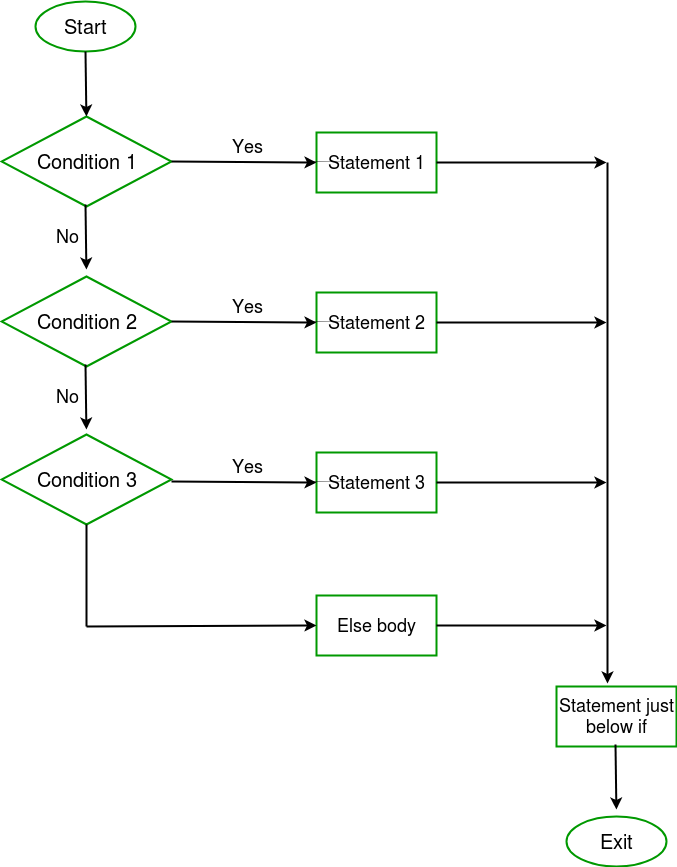Example:

## C

 `// C program to illustrate nested-if statement``#include ` `int` `main() {``    ``int` `i = 20;`` ` `    ``if` `(i == 10)``        ``printf``(``"i is 10"``);``    ``else` `if` `(i == 15)``        ``printf``(``"i is 15"``);``    ``else` `if` `(i == 20)``        ``printf``(``"i is 20"``);``    ``else``        ``printf``(``"i is not present"``);``}`

## C++

 `// C++ program to illustrate if-else-if ladder``#include``using` `namespace` `std;` `int` `main()``{``    ``int` `i = 20;`` ` `    ``if` `(i == 10)``        ``cout<<``"i is 10"``;``    ``else` `if` `(i == 15)``        ``cout<<``"i is 15"``;``    ``else` `if` `(i == 20)``        ``cout<<``"i is 20"``;``    ``else``        ``cout<<``"i is not present"``;``}`
Output:
`i is 20`

Jump Statements in C/C++

These statements are used in C orC++ for the unconditional flow of control throughout the functions in a program. They support four types of jump statements:

1. C break: This loop control statement is used to terminate the loop. As soon as the break statement is encountered from within a loop, the loop iterations stop there, and control returns from the loop immediately to the first statement after the loop.
Syntax:

`break;`
1. Basically, break statements are used in situations when we are not sure about the actual number of iterations for the loop or we want to terminate the loop based on some condition.1. Example:

## C

 `// C program to illustrate``// Linear Search` `#include ` `void` `findElement(``int` `arr[], ``int` `size, ``int` `key)``{``    ``// loop to traverse array and search for key``    ``for` `(``int` `i = 0; i < size; i++) {``        ``if` `(arr[i] == key) {``            ``printf``(``"Element found at position: %d"``, (i + 1));``            ``break``;``        ``}``    ``}``}` `int` `main() {``    ``int` `arr[] = { 1, 2, 3, 4, 5, 6 };``    ` `    ``// no of elements``    ``int` `n = 6; ``    ` `    ``// key to be searched``    ``int` `key = 3;` `    ``// Calling function to find the key``    ``findElement(arr, n, key);` `    ``return` `0;``}`

## C++

 `// CPP program to illustrate``// Linear Search``#include ``using` `namespace` `std;` `void` `findElement(``int` `arr[], ``int` `size, ``int` `key)``{``    ``// loop to traverse array and search for key``    ``for` `(``int` `i = 0; i < size; i++) {``        ``if` `(arr[i] == key) {``            ``cout << ``"Element found at position: "` `<< (i + 1);``            ``break``;``        ``}``    ``}``}` `// Driver program to test above function``int` `main()``{``    ``int` `arr[] = { 1, 2, 3, 4, 5, 6 };``    ``int` `n = 6; ``// no of elements``    ``int` `key = 3; ``// key to be searched` `    ``// Calling function to find the key``    ``findElement(arr, n, key);` `    ``return` `0;``}`
1.
Output:
`Element found at position: 3`

1.
2. C continues: This loop control statement is just like the break statement. The continue statement is opposite to that of the break statement, instead of terminating the loop, it forces to execute the next iteration of the loop.
As the name suggests the continue statement forces the loop to continue or execute the next iteration. When the continue statement is executed in the loop, the code inside the loop following the continue statement will be skipped and the next iteration of the loop will begin.
Syntax:

`continue;`
1.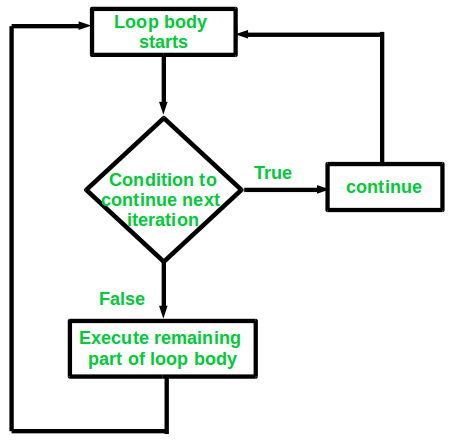1. Example:

## C

 `// C program to explain the use``// of continue statement``#include ` `int` `main() {``    ``// loop from 1 to 10``    ``for` `(``int` `i = 1; i <= 10; i++) {` `        ``// If i is equals to 6,``        ``// continue to next iteration``        ``// without printing``        ``if` `(i == 6)``            ``continue``;` `        ``else``            ``// otherwise print the value of i``            ``printf``(``"%d "``, i);``    ``}` `    ``return` `0;``}`

## C++

 `// C++ program to explain the use``// of continue statement` `#include ``using` `namespace` `std;` `int` `main()``{``    ``// loop from 1 to 10``    ``for` `(``int` `i = 1; i <= 10; i++) {` `        ``// If i is equals to 6,``        ``// continue to next iteration``        ``// without printing``        ``if` `(i == 6)``            ``continue``;` `        ``else``            ``// otherwise print the value of i``            ``cout << i << ``" "``;``    ``}` `    ``return` `0;``}`
1.
Output:
`1 2 3 4 5 7 8 9 10`

1.

If you create a variable in if-else in C/C++, it will be local to that if/else block only. You can use global variables inside the if/else block. If the name of the variable you created in if/else is as same as any global variable then priority will be given to `local variable`.

## C++

 `#include``using` `namespace` `std;` `int` `main(){``    ``int` `gfg=0; ``// local variable for main``    ``cout<<``"Before if-else block "``<

## C

 `#include ` `int` `main() {` `      ``int` `gfg=0; ``// local variable for main``    ``printf``(``"Before if-else block %d\n"``,gfg);``    ``if``(1){``        ``int` `gfg = 100; ``// new local variable of if block``        ``printf``(``"if block %d\n"``,gfg);``    ``}``    ``printf``(``"After if block %d"``,gfg);``  ``return` `0;``}`
1. C goto: The goto statement in C/C++ also referred to as unconditional jump statement can be used to jump from one point to another within a function.
Syntax

```Syntax1      |   Syntax2
----------------------------
goto label;  |    label:
.            |    .
.            |    .
.            |    .
label:       |    goto label;```
1. In the above syntax, the first line tells the compiler to go to or jump to the statement marked as a label. Here label is a user-defined identifier that indicates the target statement. The statement immediately followed after ‘label:’ is the destination statement. The ‘label:’ can also appear before the ‘goto label;’ statement in the above syntax.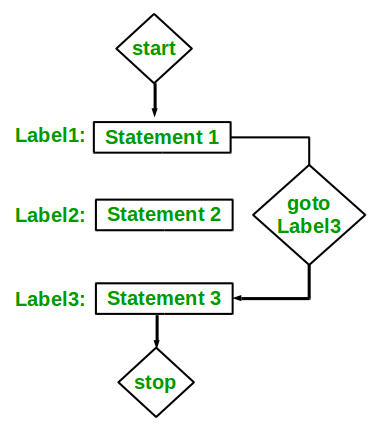1. Below are some examples of how to use goto statement:
Examples:

## C

 `// C program to print numbers``// from 1 to 10 using goto statement``#include ` `// function to print numbers from 1 to 10``void` `printNumbers()``{``    ``int` `n = 1;``label:``    ``printf``(``"%d "``,n);``    ``n++;``    ``if` `(n <= 10)``        ``goto` `label;``}` `// Driver program to test above function``int` `main() {``    ``printNumbers();``    ``return` `0;``}`

## C++

 `// C++ program to print numbers``// from 1 to 10 using goto statement``#include ``using` `namespace` `std;` `// function to print numbers from 1 to 10``void` `printNumbers()``{``    ``int` `n = 1;``label:``    ``cout << n << ``" "``;``    ``n++;``    ``if` `(n <= 10)``        ``goto` `label;``}` `// Driver program to test above function``int` `main()``{``    ``printNumbers();``    ``return` `0;``}`
1.
Output:
`1 2 3 4 5 6 7 8 9 10`

1.
2. C return: The return in C or C++ returns the flow of the execution to the function from where it is called. This statement does not mandatorily need any conditional statements. As soon as the statement is executed, the flow of the program stops immediately and return the control from where it was called. The return statement may or may not return anything for a void function, but for a non-void function, a return value is must be returned.
Syntax:

`return[expression];`
1. Example:

## C

 `// C code to illustrate return``// statement``#include ` `// non-void return type``// function to calculate sum``int` `SUM(``int` `a, ``int` `b)``{``    ``int` `s1 = a + b;``    ``return` `s1;``}` `// returns void``// function to print``void` `Print(``int` `s2)``{``    ``printf``(``"The sum is %d"``, s2);``    ``return``;``}` `int` `main()``{``    ``int` `num1 = 10;``    ``int` `num2 = 10;``    ``int` `sum_of = SUM(num1, num2);``    ``Print(sum_of);``    ``return` `0;``}`

## C++

 `// C++ code to illustrate return``// statement``#include ``using` `namespace` `std;` `// non-void return type``// function to calculate sum``int` `SUM(``int` `a, ``int` `b)``{``    ``int` `s1 = a + b;``    ``return` `s1;``}` `// returns void``// function to print``void` `Print(``int` `s2)``{``    ``cout << ``"The sum is "``<< s2;``    ``return``;``}` `int` `main()``{``    ``int` `num1 = 10;``    ``int` `num2 = 10;``    ``int` `sum_of = SUM(num1, num2);``    ``Print(sum_of);``    ``return` `0;``}`
1.
Output:
`The sum is 20`

1.

?list=PLqM7alHXFySGg6GSRmE2INI4k8fPH5qVB

This article is contributed by Harsh Agarwal. If you like GeeksforGeeks and would like to contribute, you can also write an article using write.geeksforgeeks.org or mail your article to review-team@geeksforgeeks.org. See your article appearing on the GeeksforGeeks main page and help other Geeks.